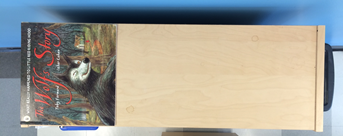# Multi-Digit Multiplication

## Objective

Solve multiplicative comparison problems with a smaller unknown, including cases that involve difficult language.

## Common Core Standards

### Core Standards

?

• 4.OA.A.1 — Interpret a multiplication equation as a comparison, e.g., interpret 35 = 5 × 7 as a statement that 35 is 5 times as many as 7 and 7 times as many as 5. Represent verbal statements of multiplicative comparisons as multiplication equations.

• 4.OA.A.2 — Multiply or divide to solve word problems involving multiplicative comparison, e.g., by using drawings and equations with a symbol for the unknown number to represent the problem, distinguishing multiplicative comparison from additive comparison.

?

• 3.OA.A.1

• 3.OA.A.3

## Criteria for Success

?

1. Solve multiplicative comparison word problems with a smaller unknown (MP.4).
2. Write an equation to represent a multiplicative comparison word problem with a smaller unknown, using a letter to represent the unknown (MP.2).
3. Solve multiplicative comparison word problems with a smaller unknown with more difficult language (e.g., “three times less”) (MP.4).

## Tips for Teachers

?

Similar to Lesson 1, while the primary focus of this lesson is on multiplicative comparison problems with a smaller unknown, the Problem Set and Homework will also spiral in comparison problems of varieties they saw in Lesson 1. This encourages students to make sense of the mathematics (MP.1).

#### Remote Learning Guidance

If you need to adapt or shorten this lesson for remote learning, we suggest prioritizing Anchor Task 2 (benefits from worked example). Find more guidance on adapting our math curriculum for remote learning here.

#### Fishtank Plus

• Problem Set
• Student Handout Editor
• Vocabulary Package

?

### Problem 1

Now, Mrs. Ingall wants to know how wide this copy of The Wolf’s Story is. Can you figure out how wide it is? (Remember, the bookshelf is 24 inches long.)### Problem 2

John has 12 coins in his pocket. John has twice as many coins as Samantha. How many coins does Samantha have? Represent the situation as an equation to solve.

### Problem 3

Ella weighs 36 pounds. Her brother, Farid, weighs four times less than her. How many pounds does Farid weigh? Represent the situation as an equation to solve.

## Problem Set & Homework

#### Discussion of Problem Set

• Look at #1. How do you know both of those expressions are correct? How can you prove that those expressions are equivalent to one another?
• Look at #3. How did you solve? How was this problem different from the others in the Problem Set?
• Look at #6. How was this problem different from the others? How did you solve? (This uses difficult language similar to Anchor Task #3.)
• Which equation is correct in #8? What made this problem more difficult? How could you change the way the relationship between toy beetles and toy spiders is described to use the language “times as many”? (Note that the Progressions generally discourage the use of fractional language to describe multiplicative relationships in Grade 4 since it’s not an expectation of the standards at Grade 4. But, because the term “half” is used far more than any other and because this is an item from a reliable source, it is included here.)

?

### Problem 1

Sanjay bought 28 granola bars, which was 4 times as many granola bars as Luke bought. Which equation can be used to find g, the number of granola bars Luke bought?

A. $28\times4=g$

B. $28+4=g$

C. $g\div4=28$

D. $28\div4=g$

### Problem 2

Vanessa has 7 times more money than Jared does. Vanessa has \$56. How much money does Jared have?

### Problem 3

Josh has 8 cookies. Sebastian has 2 times as many cookies as Josh. How many cookies does Sebastian have?

### Mastery Response

?